# How to Prove Cassini's Identity

Instructor: Gerald Lemay

Gerald has taught engineering, math and science and has a doctorate in electrical engineering.

In this lesson, individual Fibonacci numbers are related by the Cassini identity. We clarify what this identity means and show how to prove it using the method of induction.

## Cassini's Identity

In normal everyday language, ''identity'' is the who, what, where, when and why about a person. For example, Cassini's identity: Giovanni Domenico Cassini, an Italian astronomer and mathematician who lived from 1625 to 1712, famous for the discovery of the ring divisions of the planet Saturn and for the math identity which bears his name.

The topic for this lesson is a math identity. In math, an identity states something is equal to something else. It's okay to think of a math identity as an equation with one or more variables.

In this lesson we explore Cassini's (math) identity.

## Cassini and the Fibonacci Numbers

Before we can understand and prove Cassini's identity, we need to review Fibonacci numbers. By the way, the Italian mathematician, Leonardo Fibonacci, lived 4 centuries before Cassini.

Take the numbers 0 and 1. Add 1 to 0 and we get 1. Ignoring the zero, we have the sequence 1, 1.

Add the last number (1) to the previous number (1) and we get 1 + 1 = 2. Now the sequence is 1, 1, 2.

Add the last number (2) to the previous number (1) and we get 2 + 1 = 3. The sequence is 1, 1, 2, 3.

If we continue this process, the sequence becomes 1, 1, 2, 3, 5, 8, 13, 21, … These are the Fibonacci numbers.

It's nice to keep track of where we are in the sequence. We can use intergers 0, 1, 2, 3, …

 0 1 2 3 4 5 6 7 F 1 1 2 3 5 8 13 21

F is the Fibonacci number. Thus, F1 is 1 and F4 is 5. What is F6? Right, 13.

Cassini brilliantly observed: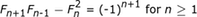This is the Cassini identity.

What is this saying? First of all, the variable in this identity is n and the values it can have are integers starting at 1. Thus, n can be 1, 2, 3, …

Looking at the right-hand side of Cassini's identity, what happens when we raise (-1) to the n + 1 power? It all depends on the value of n. For example, if n is an odd number, like 1, 3, 5, … then (-1)n + 1 = +1. You can check with n = 1 which gives (-1)1 + 1 = (-1)2 = +1. How about even numbers for n like 0, 2, 4, …. Right! We get -1.

Delving a little deeper, let's verify for a particular value of n ≥ 1. How about n = 1? Okay, for n = 1,

• Fn + 1 is F1 + 1 which is F2 which is 2 (from the table)
• Fn - 1 is F1 - 1 is F0 which is 1
• Fn is F1 which is 1

Substituting into the left-hand side of Cassini's identity:How about the right-hand side?

• (-1)n + 1 is (-1)1 + 1 (-1)2 which is +1

Substituting: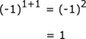Left-hand side equals the right-hand side. Thus, we have verified the identity for n = 1.

Just for fun, take a moment to verify the identity for n = 6.

Here are the details. For the left-hand side:and for the right-hand side: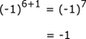Again, left-hand side equals the right-hand side!

Instead of verifying for all the other values of n (which would take forever), we use a really nice proof method.

## Proving Inductively

A proof by induction has the following steps:

1. verify the identity for n = 1

2. assume the identity is true for n = k

3. use the assumption and verify the identity for n = k + 1

4. explain and make a conclusion:

• if we backtracked to n = 1 (verified in step 1), this n = 1 could be our k
• the identity was verified for n = k + 1 provided n = k was true. Therefore, the identity is verified for all integer values of n ≥ 1. And the proof is done!

Not so bad! We've already done step 1 verifying for n = 1. On to step 2.

Replace n with k: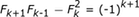and assume this to be true.

In step 3, we go to the next value of n. After n = k we have n = k + 1.

Replacing n with k + 1: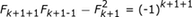and simplifying: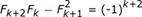The next portion of step 3 is to figure out a way to use the assumption.

We could start by replacing Fk + 2.

On the left-hand side: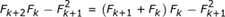We used Fibonacci where Fk + 2 is the sum of the two previous numbers, Fk + 1 and Fk.

Continuing with the left-hand side, we expand: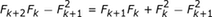Almost there! The last term, (Fk + 1 )2 becomes

To unlock this lesson you must be a Study.com Member.

### Register to view this lesson

Are you a student or a teacher?

### Unlock Your Education

#### See for yourself why 30 million people use Study.com

##### Become a Study.com member and start learning now.
Back
What teachers are saying about Study.com

### Earning College Credit

Did you know… We have over 160 college courses that prepare you to earn credit by exam that is accepted by over 1,500 colleges and universities. You can test out of the first two years of college and save thousands off your degree. Anyone can earn credit-by-exam regardless of age or education level.